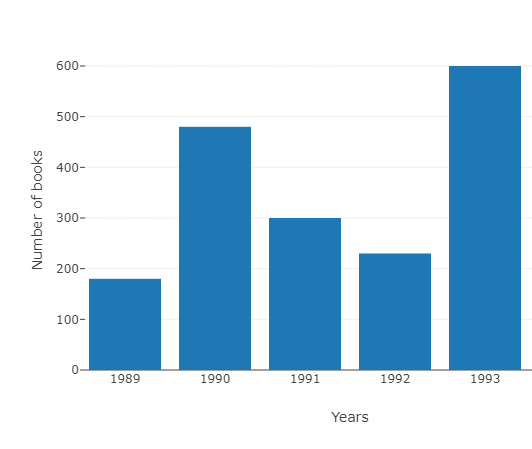# Read the bar graph $(Fig\ 3.4)$ which shows the number of books sold by a bookstore during five consecutive years and answer the following questions:$(i)$. About how many books were sold in 1989? 1990? 1992?$(ii)$. In which year were about 475 books sold? About 225 books sold?$(iii)$. In which years were fewer than 250 books sold?$(iv)$. Can you explain how you would estimate the number of books sold in 1989?"

To do:

We have to read the bar graph which shows the number of books sold by a bookstore during five consecutive years and answer the given questions.

Solution:

(i) In 1989, about 175 books were sold. In 1990, about 475 books were sold. In 1992, about 225 books were sold.

(ii) In 1990 about 475 books were sold and in 1992, about 225 books were sold.

(iii) In 1989 and 1992 fewer than 250 books were sold.

(iv) From the graph, we can conclude that 175 books were sold in 1989. This can be estimated by drawing a line from the top of the 1989 bar to the y-axis.